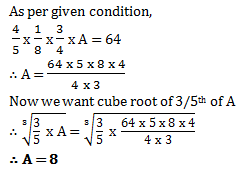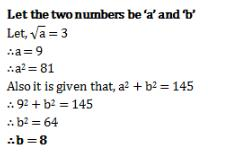Courses

# Test: Squares And Square Roots- 2

## 20 Questions MCQ Test Mathematics (Maths) Class 8 | Test: Squares And Square Roots- 2

Description
This mock test of Test: Squares And Square Roots- 2 for Quant helps you for every Quant entrance exam. This contains 20 Multiple Choice Questions for Quant Test: Squares And Square Roots- 2 (mcq) to study with solutions a complete question bank. The solved questions answers in this Test: Squares And Square Roots- 2 quiz give you a good mix of easy questions and tough questions. Quant students definitely take this Test: Squares And Square Roots- 2 exercise for a better result in the exam. You can find other Test: Squares And Square Roots- 2 extra questions, long questions & short questions for Quant on EduRev as well by searching above.
QUESTION: 1

### Four-fifth of one-eighth of 3/4th of A is 64. What is the cube root of 3/5th of A?

Solution:QUESTION: 2

### Find the least number that must be subtracted from 5607 so as to get a perfect square.

Solution:
Square of 75 is 5625
square of 74 is 5476
so use must get 5476
5607 - x is 5476
x is 131
square root of 5476 is 74
QUESTION: 3

### What will be the number of zeros in the square of 60?

Solution:
QUESTION: 4

How many natural numbers lie between 92 and 102?

Solution:

Between 92 and 102
Here, n = 9 and n + 1 = 10
∴ Natural number between 92 and 102 are (2 × n) or 2 x 9, i.e. 18.

QUESTION: 5

Find the square of 39.

Solution:

(40-1)= 1600+1-80 = 1521

QUESTION: 6

Without adding, find the sum. 1 + 3 + 5 + 7 + 9 + 11 + 13

Solution:
QUESTION: 7

Find the square root of 729.

Solution:
QUESTION: 8

Sum of squares of two numbers is 145. If square root of one number is 3, find the other number.

Solution:QUESTION: 9

If a number has 1 or 9 in the unit’s place, then it’s square ends in ________.

Solution:
QUESTION: 10

None of the square numbers end with _________ at unit’s place.

Solution:
QUESTION: 11

________ is the inverse operation of square.

Solution:
QUESTION: 12

Find the perfect square numbers between 50 and 60.

Solution:

Since we have 72=49 and 82=64.There is no perfect square between 50 and 60.

QUESTION: 13

What is the least perfect square which is divisible by 2, 4 and 6?

Solution:
QUESTION: 14

2025 plants are to be planted in a garden in such a way that each row contains as many plants as the number of rows. Find the number of plants in each row.

Solution:
QUESTION: 15

Find the greatest 4-digit number which is a perfect square.

Solution:

By division method we find the root of 9999 and we get the remainder 198.So subtracting 198 from 9999 we get 9801 as the greatest 4-digit perfect square.

QUESTION: 16

What will be the number of zeros in the square of 400?

Solution:
QUESTION: 17

What is smallest number with which 5400 may be multiplied so that the product is perfect cube?

Solution:
QUESTION: 18

The largest perfect square between 4 and 50 is

Solution:
QUESTION: 19

Without adding,find the sum of 1+3+5+7+9+11+13+15+17+19

Solution:
QUESTION: 20

Find the square root of 529.

Solution: